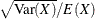# The TTEST Procedure

#### Coefficient of Variation

The coefficient of variation (abbreviated CV) of the distribution of a random variable X is the ratio of the standard deviation to the (arithmetic) mean, or. Conceptually, it is a measure of the variability of X expressed in units corresponding to the mean of X.

For lognormal data, the CV is the natural measure of variability (rather than the standard deviation) because the CV is invariant to multiplication of a lognormal variable by a constant. For a two-independent-sample design, the assumption of equal CVs on a lognormal scale is analogous to the assumption of equal variances on the normal scale. When the CVs of two independent samples of lognormal data are assumed equal, the pooled estimate of variability is used.